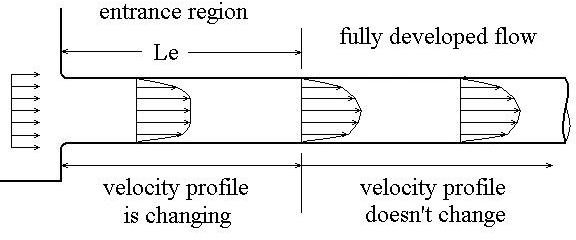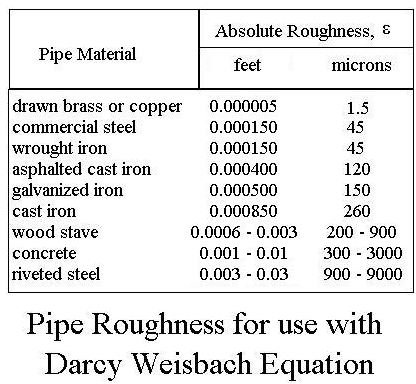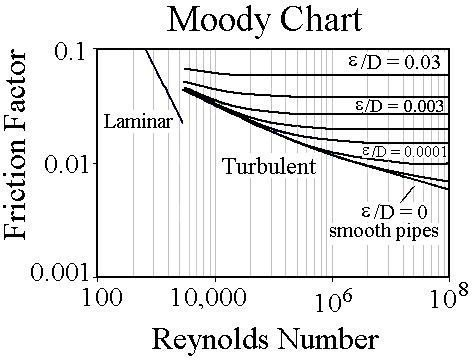# Use the Friction Factor to Calculate Frictional Head Loss (Pressure Drop) for Pipe Flow

## Introduction

Pipe flow under pressure is used for a lot of purposes. Energy input to the gas or liquid is needed to make it flow through the pipe or conduit. This energy input is needed because there is frictional energy loss (also called frictional head loss or frictional pressure drop) due to the friction between the fluid and the pipe wall and internal friction within the fluid. The Darcy Weisbach Equation, which will be discussed in this article, is commonly used for a variety of calculations involving frictional head loss, pipe diameter, flow rate or velocity, and several other parameters. The friction factor, which is used in the Darcy Weisbach equation, depends upon the Reynolds number and the pipe roughness.

## Fully Developed, Turbulent FlowThe Darcy Weisbach Equation applies to fully developed, turbulent pipe flow. Recall that pipe flow will be turbulent for a Reynolds number greater than 4000. Fully developed flow will be present in a pipe or conduit beyond the entrance length. The entrance length is where the velocity profile is adjusting to the constant profile that is present throughout the fully developed flow region. The diagram at the left illustrates the concept of the entrance region and fully developed flow.

Most pipe and conduit flow of gases and liquids with a viscosity similar to water will be turbulent flow. If the total pipe length is large compared to the entrance length, then the entrance effects are negligible and the total pipe length is used for calculations.

## The Darcy Weisbach Equation for Frictional Head Loss

The Darcy Weisbach Equation provides an empirical relationship among several pipe flow variables as shown here:

The equation is: hL = f (L/D)(V2/2g), wherehL = frictional head loss, ft-lb/lb

L = pipe length, ft

D = pipe diameter, ft

V = average flow velocity of fluid (= Q/A), ft/sec

g = acceleration due to gravity = 32.2 ft/sec2

f = friction factor, a dimensionless empirical factor that is a function of Reynolds Number (Re = DVρ/μ) and/or ε/D, where

ε = an empirical pipe roughness, ft

The table at the right shows some typical values for pipe roughness for common pipe materials.

## Relationship Between Frictional Head Loss and Frictional Pressure Drop

The energy loss in pipe flow due to friction can be expressed as a pressure drop instead of as a head loss. Chemical and mechanical engineers often work with pressure drop, whereas civil engineers usually work with head loss. The relationship between frictional head loss and frictional pressure drop is simply:

ΔPf = ρghL = γhL, where:

ΔPf = frictional pressure drop, lb/ft2,

hL = frictional head loss, ft-lb/lb (as noted above).

ρ = fluid density, slugs/ft3,

g = acceleration due to gravity, ft/sec2,

γ = specific weight, lb/ft3.

## The Friction Factor – Charts and EquationsThe friction factor (also sometimes called the Moody friction factor) can be determined for known values of Reynolds number and ε/D from empirically derived charts and/or equations. A commonly used chart is the Moody friction factor chart, shown in the diagram on the left. Clicking on the chart will give you a larger scale diagram, so you can see it better. This chart helps to illustrate how the friction factor, f, depends upon Reynolds number and pipe roughness/pipe diameter (ε/D). The straight line at the upper left on the diagram represents laminar flow, in which f is independent of ε/D and depends only on Re. The portion of the chart with horizontal lines is called the completely turbulent region, in which f depends only on ε/D. For the rest of the graph, the transition region, f depends upon both Re and ε/D. The dark solid line represents "smooth pipe turbulent flow", in which f depends only on Re.

There are equations available for friction factor for each of the four regions of the chart identified above as follows.

For laminar flow (Re < 2100): f = 64/Re

For the completely turbulent region: f = [1.14 + 2 log10(D/ε)]-2

For smooth pipe turbulent flow: f = 0.316/Re1/4

For the transition region: f = {-2 log10[(ε/D)/3.7 + 2.51/Re(f1/2)]}-2

Note that the last equation requires an iterative solution to find f for given values of ε/D and Re, or "solver" can be used in the Excel spreadsheet.

The Darcy Weisbach Equation relates the variable, hL, D, L, V, ε, ρ and μ. It’s typical use is to calculate hL, D, L, or V, when all of the other parameters are known. Some of these require iterative calculations.

For Excel spreadsheet templates that can be downloaded to make pipe flow/friction factor calculations, see the article, "Pipe Flow/Head Loss/Friction Factor Calculations Using Excel Spreadsheet Templates."

## References

For further information:

1. Munson, B. R., Young, D. F., & Okiishi, T. H., Fundamentals of Fluid Mechanics, 4th Ed., New York: John Wiley and Sons, Inc, 2002.

2. Darcy Weisbach equation history – https://biosystems.okstate.edu/darcy/DarcyWeisbach/Darcy-WeisbachHistory.htm

3. Source for pipe roughness values – https://www.efunda.com/formulae/fluids/roughness.cfm

## This post is part of the series: Pipe Flow Calculations

Pipe flow calculations include using Reynolds number to find if the flow is laminar flow or turbulent flow. Frictional head loss can be found using the Darcy Weisbach equation and the friction factor. The entrance length for fully developed flow can be found for turbulent flow and for laminar flow.